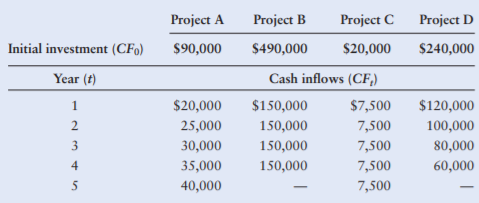### Create an Account

Already have account?

### Forgot Your Password ?

Home / Questions / Internal rate of return For each of the projects shown in the following table calculate th...

# Internal rate of return For each of the projects shown in the following table calculate the internal rate of return IRR Then indicate for each project the maximum cost of capital that the firm

Internal rate of return For each of the projects shown in the following table, calculate the internal rate of return (IRR). Then indicate, for each project, the maximum cost of capital that the firm could have and still find the IRR acceptable.Jun 03 2021 View more View Less

#### Answer (UnSolved)Get Solution